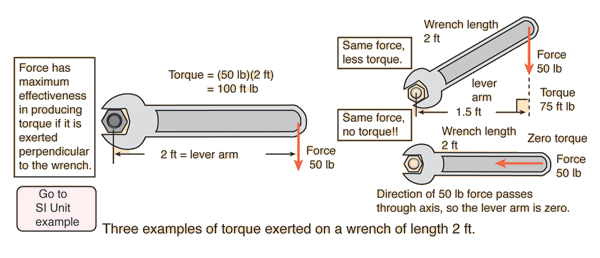# Torque

A torque is an influence which tends to change the rotational motion of an object. One way to quantify a torque is

## Torque = Force applied x lever arm

The lever arm is defined as the perpendicular distance from the axis of rotation to the line of action of the force.Torque calculation
 Torque expressions: Trigonometric Vector Product
Index

Rotation concepts

Torque concepts

 HyperPhysics***** Mechanics ***** Rotation R Nave
Go Back

# Conditions for Equilibrium

An object at equilibrium has no net influences to cause it to move, either in translation (linear motion) or rotation. The basic conditions for equilibrium are:The conditions for equilibrium are basic to the design of any load-bearing structure such as a bridge or a building since such structures must be able to maintain equilibrium under load. They are also important for the study of machines, since one must first establish equilibrium and then apply extra force or torque to produce the desired movement of the machine. The conditions of equilibrium are used to analyze the "simple machines" which are the building blocks for more complex machines.

Index

 HyperPhysics***** Mechanics ***** Rotation R Nave
Go Back

# Torque

A torque is an influence which tends to change the rotational motion of an object. One way to quantify a torque is

## Torque = Force applied x lever arm

The lever arm is defined as the perpendicular distance from the axis of rotation to the line of action of the force.

Example in U.S. common unitsOK, this is a ridiculously large wrench. But it makes the numbers easy.

Index

Rotation concepts

Torque concepts

 HyperPhysics***** Mechanics ***** Rotation R Nave
Go Back